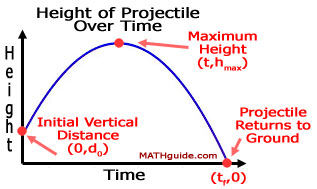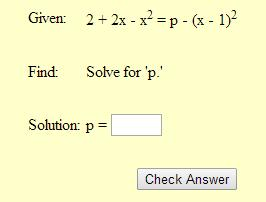Standardized Test Questions and Solutions
 Home > Lessons > Standardized Tests Search | Updated June 19th, 2020
Introduction

In this section, you will learn how to solve ACT, IAR, SAT, and PARCC problems. Here are the sections within this lesson:

 What are Standardized Tests? There are tests that assess students' readiness for college and general performance. Tests like the ACT, SAT, IAR, and PARCC are such tests. In genmeral, they measure student and school growth. These exams will cover a myriad of mathematics topics.     The sample problems below are advanced level tasks that high school students will likely encounter on a such exams. Flight of a Projectile ProblemMATHguide has this lesson within another section. Also find an activity and a quiz. Use the links below to access them.esson: Flight of a Projectileideo: Flight of a Projectileuiz: Flight of a Projectilectivity: Flight of a Projectile Picture Frame ProblemMATHguide has this lesson within another section, too. Once there, find a quiz. Use the links below to access them.ideo: Picture Frame Problemesson: Picture Frame Problemuiz: Picture Frame Problem Solve a Special Quadratic EquationThis resource will assist in learning how to solve a special type of quadratic equation.ideo: Solve a Quadratic Equationuiz: Solve a Quadratic Equation Solve for 'P' ProblemMATHguide has two resources for this PARCC exam problem. There is an instructional video and a quiz.ideo: Solving for 'P' Problemuiz: Solving for 'P' Problem Volume of an Open BoxMATHguide has a video on how to build and calculate the volume of an open box from a sheet of cardboard.ctivity: Volume of an Open Box (Static)ideo: Volume of an Open Box (Example)uiz: Volume of an Open Boxctivity: Volume of an Open Box (Dynamic)ideo: Volume of an Open Box (Dynamic) Instructional Videos Try these videos, which are closely related to the lessons above.ideo: Solve a Quadratic Equationideo: Flight of a Projectileideo: Solving for 'P' Problemideo: Volume of an Open Box (Static)ideo: Volume of an Open Box (Dynamic) Interactive Quizzes Try these quizzes, which are closely related to the lessons above.uiz: Flight of a Projectileuiz: Picture Frame Problemuiz: Solve a Quadratic Equationuiz: Solving for 'P' Problemuiz: Volume of an Open Box Related Lessons Try this lesson, which is closely related to the lessons above.esson: Operations on Polynomialsesson: Polynomial Modelsesson: Using the Quadratic Formula     See also our Puzzles and Games Page for challenging math problems.